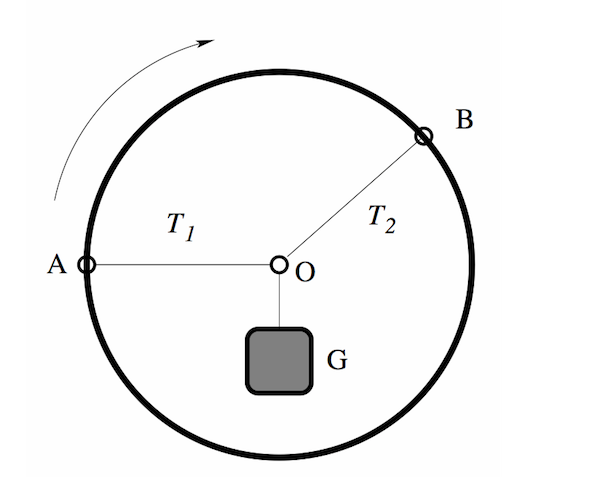# A Rigid Problem About A Rigid HoopA rigid hoop can rotate about the center. Two massless strings are attached to the hoop, one at $A$, the other at $B$. These strings are tied together at the center of the hoop at $O$, and a weight $G$ is suspended from that point. The strings have a fixed length, regardless of the tension, and the weight $G$ is only supported by the strings. Originally $OA$ is horizontal.

Now, the outer hoop will start to slowly rotate $90^\circ$ clockwise until $OA$ will become vertical, while keeping the angle between the strings constant and keeping the object static. Which of the following statements about the tensions $T_1$ and $T_2$ in the two strings is correct?

Note: This problem appeared on the 2012 F = ma exam.

×

Problem Loading...

Note Loading...

Set Loading...